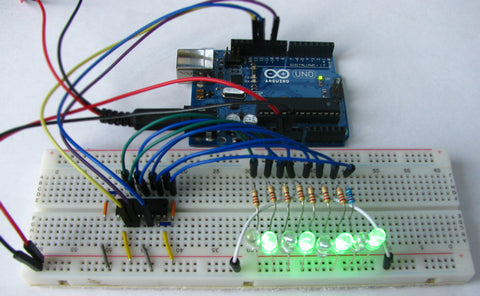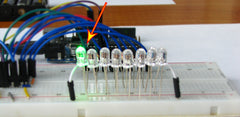# 📟 Shift register 74HC595

A shift register is a set of consecutive triggers (usually there are 8 of them). Unlike standard registers, shift supports the shift function to the right and left. (i.e. rewriting data from each previous trigger to the next one).The functionality and purpose of the shift registers are quite large. Today we will introduce one of them to the Arduino (A great way to multiply the outputs from the Arduino: occupy 3, we get 8).

Probably the most popular chip, which is such a register - is 74HC595.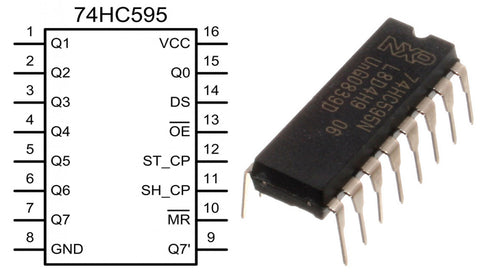- Works on the SPI interface: DS, ST_CP, SH_CP legs are control buses. Respectively: data bus (MOSI), latch (SS) and a clock line (SCK). We connect to any 3 Arduino contacts (the SPI library in the code will not be used). I have 12, 10, 13 outputs of the Arduino (standard).

- The legs Q0, Q1, ..., Q7 are the outputs of the register (bits). In order to monitor the status of each of them, we will hang each LED through each LED (with a series-connected resistor. The nominal value is from 150 to 330 Ohms)

“VCC and GND are power.” Connect to + 5v and GND.

- output Q7 `do not touch (designed for the serial connection of such registers)

- MR is a reset. Connect to + 5v (reset is not active).

- well, we attract OE to the ground (we connect to the GND contact).

It turns out, such a scheme:On the BreadBoard you can put it here, like this: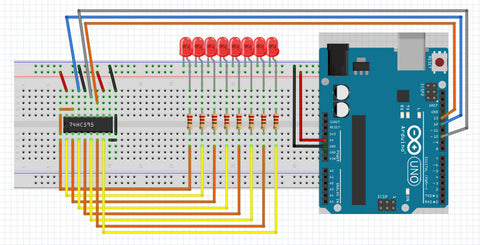Now to the code:

- As mentioned earlier, the SPI library will not be used. There is a convenient shiftOut () function.

First of all, we call our pins (clock line - clock, data - data, latch - latch):

`#define data 12#define clock 13`
`#define latch 10`

then in the void setup(), we denote them as outputs and immediately put a high level on the latch so that the register does not accept signals:

`void` `setup``(){`
`  ``pinMode``(clock, ``OUTPUT``);`
`  ``pinMode``(data, ``OUTPUT``);`
`  ``pinMode``(latch, ``OUTPUT``);`
`  ``digitalWrite``(latch, ``HIGH``);`
`}`

Now let's try to send something to the register:
- for a start, we put LOW on the latch (we start data transfer. Now the register receives signals from the Arduino).

`digitalWrite``(latch, ``LOW``);`

then we send the data (i.e., we send the byte in digital or binary form. In the binary it is simpler because each of the 8 bits is responsible for its bit in the register. It’s easier to navigate with your eyes):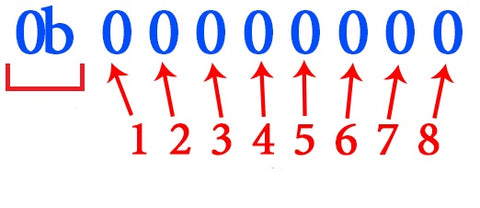To begin, send bytes 0b10000000; (the first LED should light up):

`shiftOut``(data, clock, LSBFIRST,0b10000000);`

and at the end, we set HIGH on the latch (we finish transmitting data).

`digitalWrite``(latch, ``HIGH``);`

As a result, all of our code:

`#define clock 13`
`#define data 12`
`#define latch 10`

`void` `setup``() {`
`  ``pinMode``(clock, ``OUTPUT``);`
`  ``pinMode``(data, ``OUTPUT``);`
`  ``pinMode``(latch, ``OUTPUT``);`
`  ``digitalWrite``(latch, ``HIGH``);`
`}`

`void` `loop``() {`
`  ``digitalWrite``(latch, ``LOW``);`
`  ``shiftOut``(data, clock, LSBFIRST, 0b10000000);`
`  ``digitalWrite``(latch, ``HIGH``);`
`}`

Now we load in Arduino. The result should be the following (the first LED lights up):(if your first LED does not light up, then in the shiftOut function, change LSBFIRST to MSBFIRST and everything will fall into place).
So, it turned out! I propose to create a function so that each time you do not write these 3 lines:
I will call it: sendbyte;

`void` `sendbyte(``byte` `value){`
`  ``digitalWrite``(latch, ``LOW``);`
`  ``shiftOut``(data, clock, LSBFIRST, value);`
`  ``digitalWrite``(latch, ``HIGH``);`
}

This function sends the status of all digits to the register at once. This is useful for managing the seven-segment. But to use the register as a port expander, you need to manage each bit separately (similar to the digitalWrite () function):

- We can send only full bytes to the register (8 bits - 0b00000000). If you send not 8, but 5 bits (for example 0b00000000), then the register will wait for the missing 3 bits. This means that when we want to change the state of one register bit (turn it on or off), we must, in fact, send a previously sent byte, with a change of one bit.

(P. S .: Now is a long and tedious explanation (for beginners) who are not interested, go down a little lower :);
- So, first, create a so-called (by me) database in which the state of each digit will be stored (on (HIGH) or off (LOW)). type: boolean:

boolean states;

We just had an array of variables;
Each variable in this array denotes its own bit (in zero (in a row) the state of 1 digit will be stored, the second - 3rd, and so on)
- Now we will write a function (I will call it: sendpin). It will take 2 values: the number of the category, and the level that we need to attribute to this category: high (HIGH) or low (LOW).

`void` `sendpin(``int` `pin, ``boolean` `state){`
`  ``pin--;`
`  ``states[pin]=state;`
`  `
`  ``byte` `value = 0;`
`  ``byte` `add = 1;`
`  ``for``(``int` `i=0; i<8; i++){`
`    ``if``(states[i]==``HIGH``) value+=add;`
`    ``add*=2;`
`  ``}`
`  ``digitalWrite``(latch, ``LOW``);`
`  ``shiftOut``(data, clock, LSBFIRST, value);`
`  ``digitalWrite``(latch, ``HIGH``);`
`}`

due to the fact that the score starts from zero, we have to call the first pin zero. To fix this (we will write as is (the first means the first), and the Arduino will answer one by itself), I wrote:

pin--;

Then we note the changes in the database:

states[pin]=state;

Now it is necessary to form 8 bytes of bytes and send it to the register.
- for starters, create variables:
value - the byte that will be sent. (by default it should be made zero):

byte value = 0;

add is a variable that will hold the byte of the current bit. for the first digit, it is byte 1 (0b10000000);

now we need to scroll through all 8 variables in the database and form a byte (we will do this using a for () loop:

for (int i = 0; i <8; i ++) {

}

So, every time we check the next digit in the database. If it should have a high level, then we add to value add and go to the next digit in the chain (as if moving a bit higher (to the left). That is, everything is simple in binary code: it was like this: 0b01000000; shifted one to the left and it turned out like this: 0b10000000. But in a digital form everything is different. Shifting to the left is similar to multiplying by 2 (and to the right, by the way, dividing by 2)). It turns out like this:

if (states [i] == HIGH) value + = add;

Now it only remains to send the value to the register:

digitalWrite (latch, LOW);
shiftOut (data, clock, LSBFIRST, value);
digitalWrite (latch, HIGH);

In principle, if you understand, everything is very simple.

So, let's try to turn on 2, 4, 6, and 8 bits separately (we will write our function in a loop 4 times):

sendpin (2, HIGH);
sendpin (4, HIGH);
sendpin (6, HIGH);
sendpin (8, HIGH);

And by the way, in setup, you need to clear the register (send 0).

You can even create such a function:

void cleanreg () {
for (int i = 0; i <8; i ++) states [i] = LOW;
digitalWrite (latch, LOW);
shiftOut (data, clock, LSBFIRST, 0);
digitalWrite (latch, HIGH);
}

In general, the result is:

﻿